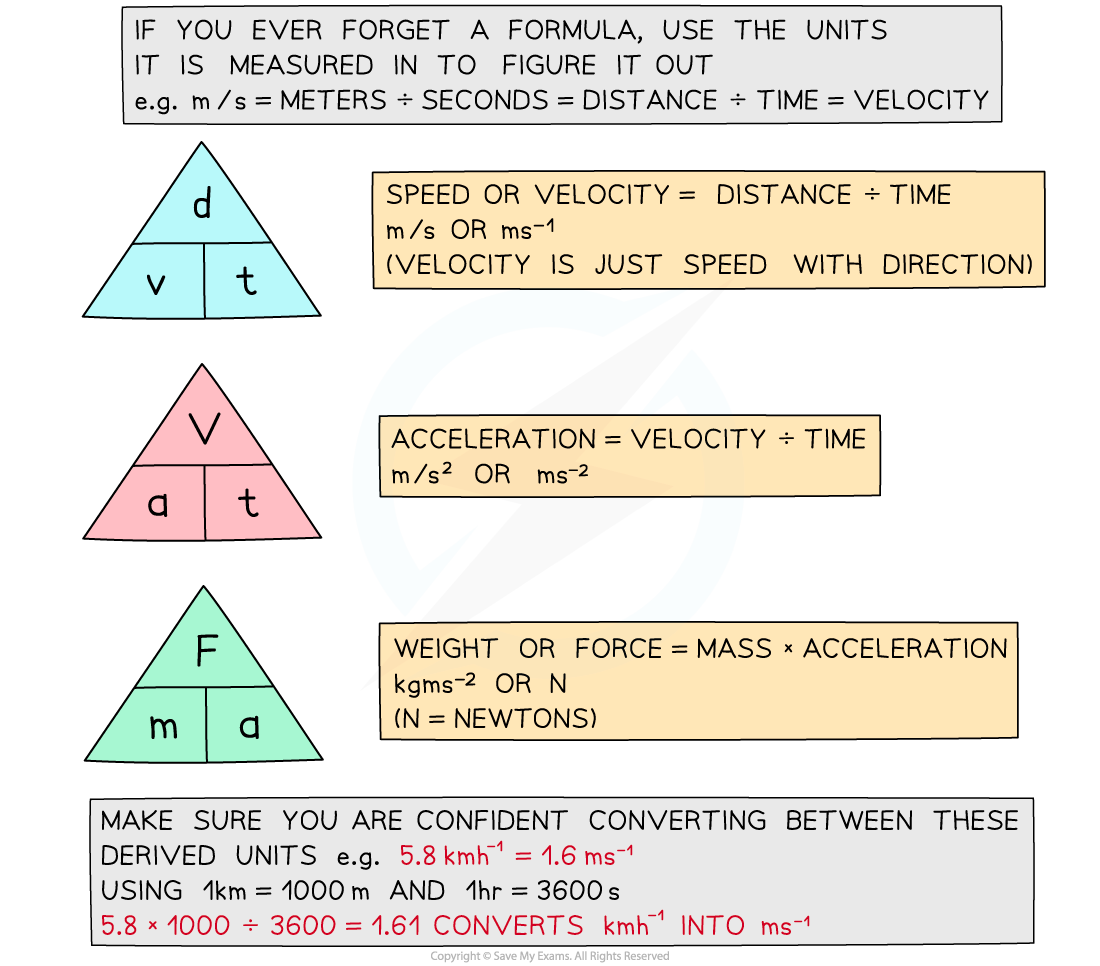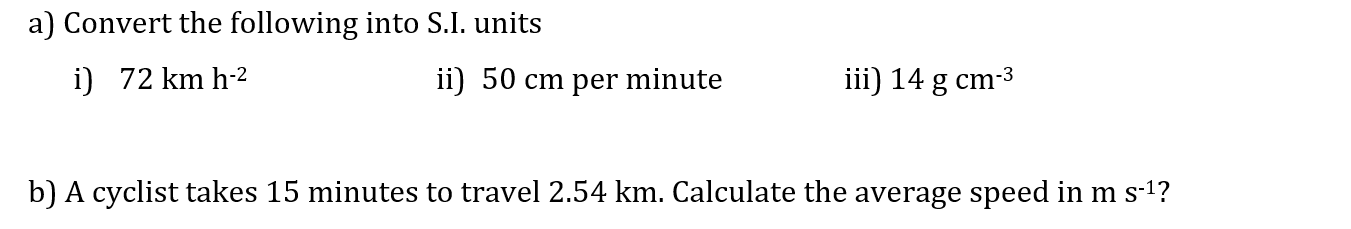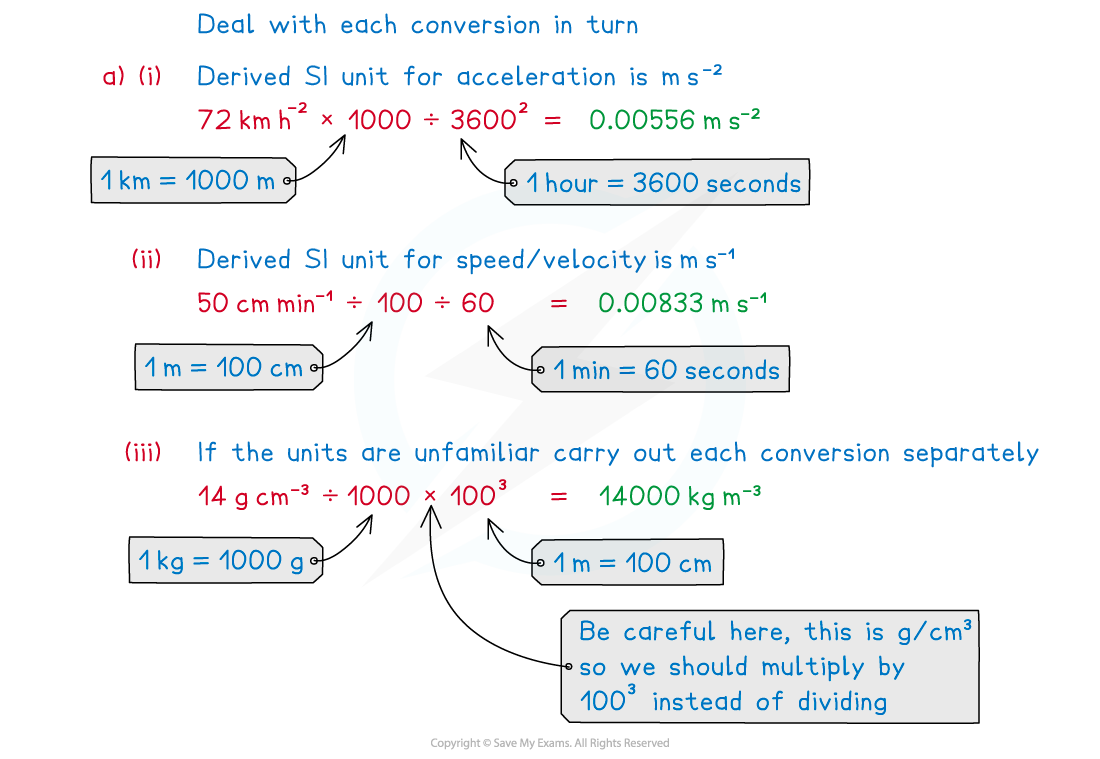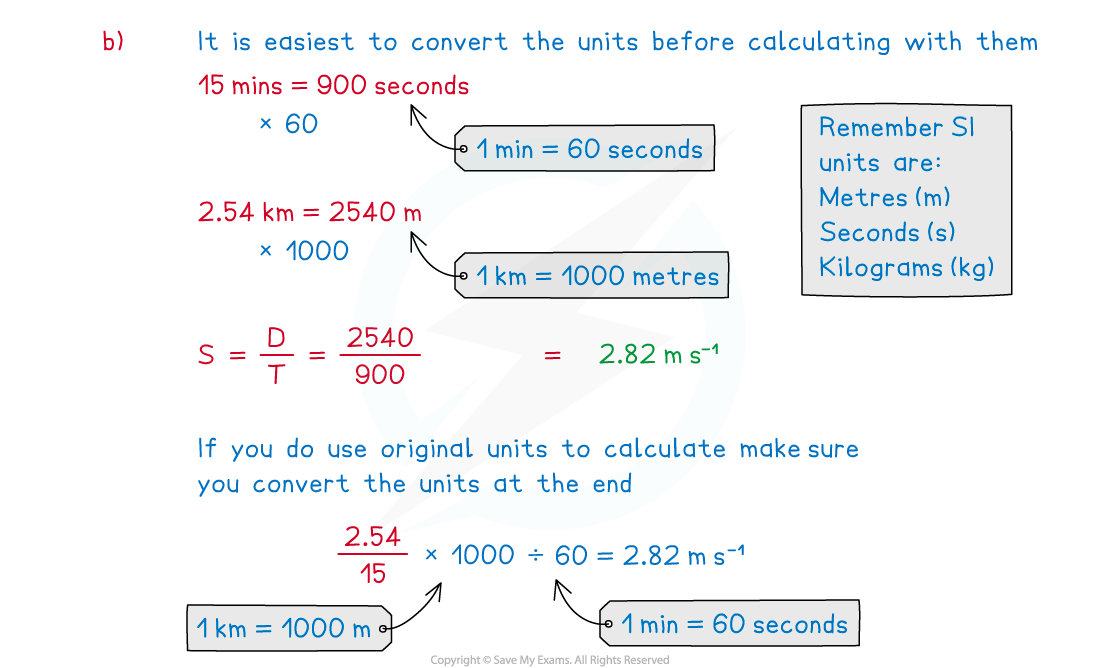# CIE A Level Maths: Mechanics复习笔记1.1.3 Derived Units

### Derived Units

#### What are derived units?

• Derived units for quantities such as velocity, acceleration and force etc. are combinations of S.I. units
• Speed or Velocity = distance ÷ time m/s or m s-1 (Velocity is just speed with direction)
• Acceleration = velocity ÷ time = ms-1 /s = m/s2 or m s-2
• Weight or Force = mass x acceleration = kg m s-2 or N (N = Newtons)#### Worked Examplea) Convert the following into S.I. units

i) 72 km h-2                    ii)   50 cm per minute                   iii)   14 g cm-3b) A cyclist takes 15 minutes to travel 2.54 km. Calculate the average speed in m s-1?#### Exam Tip

• Watch out for exam questions using non S.I. units such as feet and inches or miles per hour.
• Make sure you are confident converting between these derived units,
• Remember speed is velocity without direction and weight is a force measured in Newtons, not a mass measured in kg.
• If you ever forget a formula, use the units it is measured in to figure it out,
• e.g. m/s = metres ÷ seconds = distance ÷ time = velocity.